#### You may also like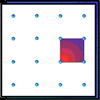### Geoboards

This practical challenge invites you to investigate the different squares you can make on a square geoboard or pegboard.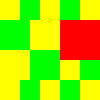### Tiles on a Patio

How many ways can you find of tiling the square patio, using square tiles of different sizes?### Pebbles

Place four pebbles on the sand in the form of a square. Keep adding as few pebbles as necessary to double the area. How many extra pebbles are added each time?

# Cover the Tray

##### Age 7 to 11 Challenge Level: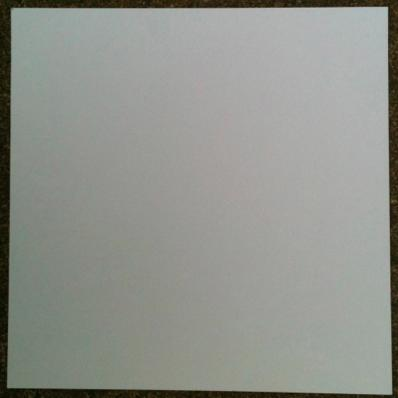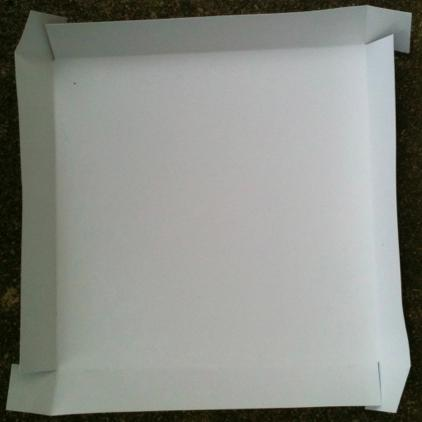Please note: this problem uses whole numbers of centimetres throughout.

Draw the same smaller square in each corner of the card.
Cut one edge of each of these squares so that you can fold the card up to make a tray.
(See the pictures above.)

We are going to cover the tray.  The covering goes over all that can be seen, so it is just the square base underneath that remains uncovered.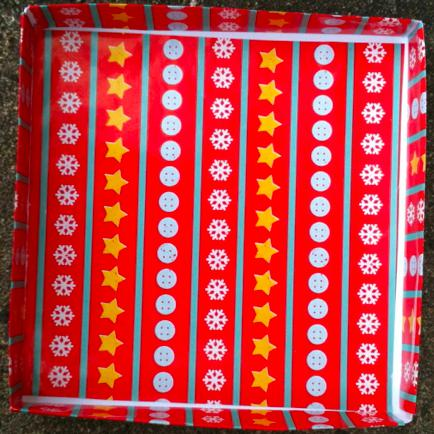First challenge
If the card is 18cm x 18cm and the amount of wrapping paper used is 432cm$^2$, what are the dimensions of the base of this tray and what size are the square corners that need to be cut?

Second challenge
The amount of wrapping paper used is 420cm$^2$.
What possible squares of card can you start with and what size corners can you use?

Third challenge
The amount of wrapping paper used can be between 400cm$^2$ and 499cm$^2$.
What possible squares of card and corners can you find?
Can you find a system that helps you get them all?

This problem featured in the final of the Young Mathematicians' Award.